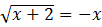### Sample Problem

Solvex =

#### Solutionx+2++1=3-x

-2x =x + 2 = x2

x2-x-2=0

x = 2 or x = -1

Testing x = 2  proves that it is an incorrect solution, so x = -1